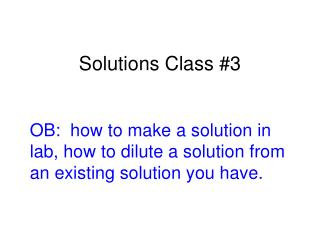DownloadDownload PresentationSolutions Class #3

# Solutions Class #3

Download Presentation## Solutions Class #3

- - - - - - - - - - - - - - - - - - - - - - - - - - - E N D - - - - - - - - - - - - - - - - - - - - - - - - - - -
##### Presentation Transcript

1. Solutions Class #3 OB: how to make a solution in lab, how to dilute a solution from an existing solution you have.

2. How to properly mix a solution How would you mix up a 1.00 molar NaCl aqueous solution? Right Wrong Put 58 g NaCl into your beaker, then fill up to 1000 mL Add 58 g NaCl to 1.0 liters of water THENFill to1000 mL line NaCl first

3. You have an aqueous solution of magnesium chloride of 1505 mL, and it has 0.50 moles solute dissolved. What is its concentration?

4. You have an aqueous solution of magnesium chloride of 1505 mL, and it has 0.50 moles solute dissolved. What is its concentration? # moles soluteLiters solution 0.50 moles MgCl21.505 Liters M = = = 0.33 M MgCl2(AQ)(2 SF)

5. When 3.20 moles copper (I) chloride is dissolved into 8.50 liters solution, what is the solution’s concentration?

6. When 3.20 moles copper (I) chloride is dissolved into 8.50 liters solution, what is the solution’s concentration? # moles soluteLiters solution 3.20 moles CuCl8.50 Liters M = = = 0.376 M CuCl(AQ)(3 SF)

7. Let’s assume that you have some strong salty water, and you know it’s molarity to be 5.50 Molar. You’d like to make a less concentrated solution of 100. mL of just 1.00 molarity. You could mix some of it with some deionized water, but HOW MUCH of the original “stock” solution and how much water, that is the question! There is a formula for dilution of a solution: M1V1 = M2V2M for molarity, V for volume

8. How do you prepare a 100. mL NaCl(AQ) solution of 1.00 molarity from a stock solution of 5.50 M? M1V1 = M2V2

9. How do you prepare a 100. mL NaCl(AQ) solution of 1.00 molarity from a stock solution of 5.50 M? M1V1 = M2V2 (5.50 M)( X mL) = (1.00 M)(100. mL) x mL = (1.00 M)(100. mL)5.50 M x = 18.2 mL stock solution(3 SF) But is that (?) the answer???

10. Short answer, nope. It’s not enough. Let’s think. We want a 100. mL solution, this math tells us HOW MUCH STOCK solution to start with. So… Then, fill up to exactly 100. mL with water. (Swirl, gargle, spit.) water Stock solution First, put your 18.2 mL STOCK SOLUTION into a new beaker.

11. When the school buys hydrochloric acid, it’s cheaper to ship us a single gallon of super duper strong acid, than to mail us 12 gallons of weaker acid. Plus, with the strong stuff, we can make up our own solutions, of exactly the strength we need for you kiddies to learn with. We buy 12.0 M HCl(AQ). How would you mix up a 2.00 liter hydrochloric acid solution of 5.25 M?

12. We buy 12.0 M HCl(AQ). How would you mix up a 2.00 liter hydrochloric acid solution of 5.25 M? M1V1 = M2V2 (12.0 M)(x L) = (5.25 M)(2.00 L) x L = (5.25 M)(2.00 L)12.0 M X = 0.875 L stock solution

13. 13

14. To mix this solution up, you would first take exactly 0.875 Liters of stock HCl(AQ) (that is 875 mL), and put it into a big beaker, then you’d pour in enough water to dilute it to 2.00 liters (that is 2000 mL). 875 mL stock + 1125 mL water = 2000 mL new solution

15. CO2 in Soda Lab During this lab we will measure some Coca Cola soda carefully. We are going to try to determine in a given volume of soda, how many grams of carbon dioxide are dissolved into it. Then, with that information, we will calculate the % composition by mass of carbon dioxide in Coke, and then, the Molarity of this CO2(G) solution. We will not drink this soda. We will not let the soda boil, or even get hotter than 50°C. We will carefully clean up and get the sugar off of everything, including the beakers, thermometers, tables, etc. The cans will need to be rinsed out before being returned to the teacher (I paid, I’ll give my son the nickels from the cans).